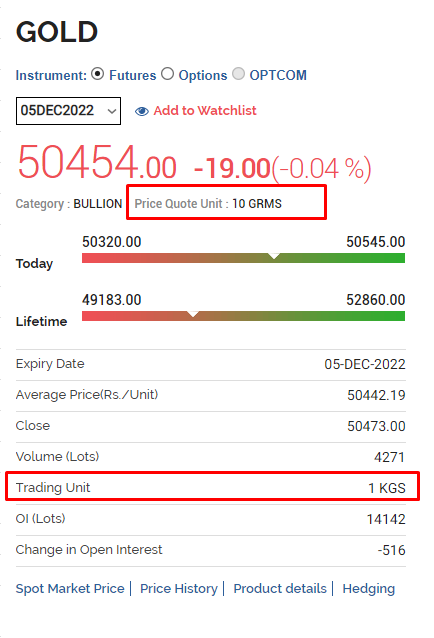# what is value of multiplier in options

I want to calculate real time profit and loss in my application for options that i bought, i am using below formula to calculate PNL

pnl = (sellvalue - buyvalue) + (quantity * ltp * multiplier)

It shows the profit and loss accurately

What is the use of multiplier and what are the different values for multiplier in options .Could any one explain with some examples .
Tagged:
• `multiplier` means lot size of that particular contract. Go through the positions response attribute doc here.
• edited October 2022
So lets say i bought 2400 quantity of Nifty 50 option, and the lot size for Nifty 50 is (50) , so when i fetch positions, i will get quantity as 48 and multiplier as 50, right? Is it mandatory to use multiplier to calculate profit and loss
• Yes, each position will have multiplier attribute just use that for calculation.
• @sujith What are the different values for multiplier in options .Could you explain with some examples .
If possible can we have a call for it
• edited October 2022
I am asking this because i bought 100 quantities of Nifty 50 option but in response from positions got quantity as 100 and multiplier was 1, then how can multiplier be the lot size? Because the lot size was 50 when i bought the option
• edited October 2022
@RahulS The multiplier field is needed for currency and commodity F&O. For all NFO instruments, you'll see the multiplier as 1.
For instance, USDINR 1 quantity on the exchange = 1000 units of USDINR. The multiplier comes in use here while calculating contract value, P&L, etc. The same is the case for commodity contracts where the trading unit and the price quote unit are different.